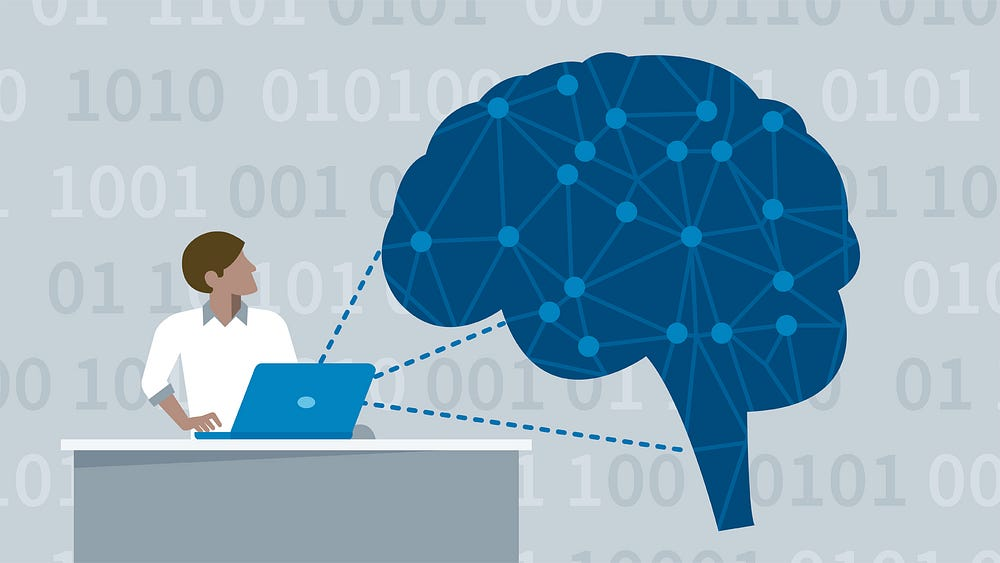# LSTM and its equations

LSTM stands for Long Short Term Memory, I myself found it difficult to directly understand LSTM without any prior knowledge of the Gates and cell state used in Long Short Term Memory neural networks so, this post is an attempt to get familier with a LSTM model which uses gates and cell state.

#### Why do we need LSTM if we have RNN?

LSTM can be used to solve problems faced by the RNN model. So, it can be used to solve:

1. Long term dependency problem in RNNs.

The heart of a LSTM network is it’s cell or say cell state which provides a bit of memory to the LSTM so it can remember the past.

i.e The cell state may remember the gender of the subject in a given input sequence so that the proper pronoun or verb can be used.

Let us consider some examples:

1. The cat which already ate ………………… was full.
2. The cats which already ate …………………. were full.
• in between the dots represents the presence of a long sentence but the subject has not changed yet.

In the first sentence “The cat” is singular so, the lstm cell must remember that feature to use “was”.

Similarly, in second example “ were” should be used for the subject “The cats”.

LSTM is made up of Gates:

In LSTM we will have 3 gates:

1) Input Gate.

2) Forget Gate.

3) Output Gate.

Gates in LSTM are the sigmoid activation functions i.e they output a value between 0 or 1 and in most of the cases it is either 0 or 1.

we use sigmoid function for gates because, we want a gate to give only positive values and should be able to give us a clear cut answer whether, we need to keep a particular feature or we need to discard that feature.

“0” means the gates are blocking everything.

“1” means gates are allowing everything to pass through it.

The equations for the gates in LSTM are:

First equation is for Input Gate which tells us that what new information we’re going to store in the cell state(that we will see below).

Second is for the forget gate which tells the information to throw away from the cell state.

Third one is for the output gate which is used to provide the activation to the final output of the lstm block at timestamp ‘t’.

### The equations for the cell state, candidate cell state and the final output:

To get the memory vector for the current timestamp (c_{t}) the candidate is calculated.

Now, from the above equation we can see that at any timestamp, our cell state knows that what it needs to forget from the previous state(i.e f_{t} * c_{t-1}) and what it needs to consider from the current timestamp (i.e i_{t} * c`_{t}).

note: * represents the element wise multiplication of the vectors.

Lastly, we filter the cell state and then it is passed through the activation function which predicts what portion should appear as the output of current lstm unit at timestamp t.

We can pass this h_{t} the output from current lstm block through the softmax layer to get the predicted output(y_{t}) from the current block.

Let’s look at a block of lstm at any timestamp {t}.

With the help of all the equations mentioned above, we can easily understand the above block or we can ourself draw the block diagram.

If anyone have suggestions please comment it below or if the post helped you, give it a clap!!.

Source: Deep Learning on Medium